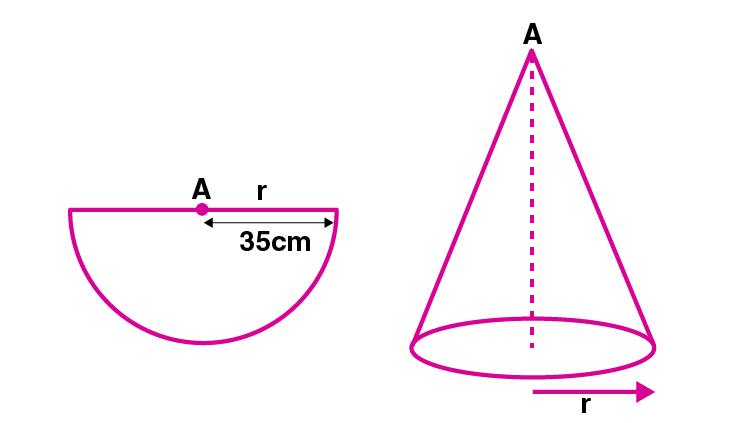Newbie

# A semi-circular lamina of radius 35 cm is folded so that the two bounding radii are joined together to form a cone. Find (i) the radius of the cone. (ii) the (lateral) surface area of the cone.

• 0

An Important Question of M.L Aggarwal book of class 10 Based on Mensuration Chapter for ICSE BOARD.
If a semi-circular lamina of a radius is folded so that the two bounding radii are joined together to form a cone. Find (i) the radius of the cone. (ii) the (lateral) surface area of the cone.
This is the Question Number 19, Exercise 17.2 of M.L Aggarwal.

Share

1.(i)Given radius of the semi circular lamina, r = 35 cm

A cone is formed by folding it.

So the slant height of the cone, = 35 cm

Let r1 be radius of cone.

Semicircular perimeter of lamina becomes the base of the cone.

r = 2r1

r = 2 r1

35 = 2 r1

r1 = 35/2= 17.5 cm

Hence the radius of the cone is 17.5 cm.

(ii) Curved surface area of the cone = r1l

= (22/7)×17.5×35

= 22×17.5×5

= 1925 cm2

Hence the lateral surface area of the cone is 1925 cm2.

• 2